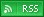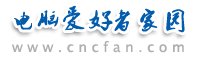电脑爱好者，提供IT资讯信息及各类编程知识文章介绍，欢迎大家来本站学习电脑知识。 最近更新 | 联系我们站内搜索：

# 不错的VBscript代码

<SCRIPT language="vbscript">
num=4
msgbox "进入页面前，请一定照着提示上说的回答，可不要乱写，不然的话会让测试不准确的!切记！现在放松一下，记住，写的时候应该完全是你的第一直觉！！！"
msgbox"这个测试源于印度的一个神秘的密教经典，当时发现它的人按照它说的做了，结果他的愿望在10分钟内就实现了!"
msgbox"这是一个非常奇妙的测试，不知道你做过没有，最好没有旁观者！我做了，非常准确，想不信邪都难!"
y1 = inputbox("1.请输入你一个异性朋友的名字","")
y2 = inputbox("2.请再输入你一个异性朋友的名字","")
t1 = inputbox("3.输入你一个同性朋友的名字","")
t2 = inputbox("4.输入你第二个同性朋友的名字","")
t3 = inputbox("5.再次输入你一个同性朋友的名字","")
m1 = inputbox("6.输入一首你喜欢的歌的名字","")
m2 = inputbox("7.输入另外一首歌的名字","")
m3 = inputbox("8.再输入一首歌的名字 请不要急躁，保持轻松的心态","")
m4 = inputbox("9.最后输入一首喜欢的歌名字","")
num = inputbox("10.输入一个你喜欢的幸运数字","")
wish = inputbox("11.许下一个你的愿望","")
num=num *4

</script>
<SCRIPT language="vbscript">
aa=""
aa=aa+"<div id=ww0 style='DISPLAY: none'><TABLE align=center border=0 width='90%'><TBODY><TR><TD> 在这个情人节里" + y1 + " 占据着最重要的位置，你对 " + y1 + " "
aa=aa+"的感觉用 " + m1 + " 这首歌来形容再好不过了。你相信这是上天安排的缘分，因为你深深的爱着"
aa=aa+" " + y1 + " "
aa=aa+"。 对于 " + y2 + " "
aa=aa+"，也许是宿命吧，虽然你也喜欢 " + y2 + " "
aa=aa+"，但是你们注定不可能在一起，就象 " + m2 + " "
aa=aa+"中唱的一样。<p> " + t1 + " "
aa=aa+"是最关心你的人，而 " + t2 + " "
aa=aa+"是最了解你的人，你们两个在一起会彼此有知己的感觉。 " + t3 + " "
aa=aa+"是你的幸运星，也许会给你带来好运气的哦~!~!~! <p align='left'> " + m3 + " "
aa=aa+"这首歌最能代表你现在的想法。 " + m4 + " "
aa=aa+"这首歌代表你对生活的感受。 把本页推荐给你的 " + cstr(num) + " "
aa=aa+"个朋友，你的愿望--- " + wish + " 就会实现，信不信由你！<br>"
aa=aa+"<br><b><font color=#FF0000>不要把这个心愿放在心里。否则这个心愿会在96小时后离开你。<br>将本页发给你的网友。<br>"
aa=aa+"让您的好友也来许愿，看看会发生什么？<br>在今后的四天里，你会收到一个令你高兴的惊喜。<br>好的东西记得与朋友分享噢！<br></font></b><br>"
aa=aa+"<font color=#276BD1>0-10 人: 你会经历一个小小的变化。<br>11-19 人: 你的生活会按照你期望的方向改进。<br> 20-30 人: 在今后4天，你至少得到5个快乐的惊喜。<br> 30 人以上:你的生活会发生惊人的变化，你的美梦将会成真。</font></p></TD></TR></TBODY></TABLE></div>"
document.write(aa)
</script>

<SCRIPT language=VBScript>
dim w,t,p,mm,c,mr,dy,tmp,vs:vs=5:dy=1:p=1:mr="<BR><BR><BR>":c="<font color=white><B>|"&mr:Set w = document.body

sub tp
m=mid(t,p,1):if m="" then c=mr
if m="`" then m="":mm=mid(mm,1,len(mm)-1)
if m="<" then av=instr(mid(t,p),">"):m=mid(t,p,av):p=p+av-1
if m="&" then av=instr(mid(t,p),";"):m=mid(t,p,av):p=p+av-1
tmp=100:if m="." then tmp=400 else if m="," then tmp=200 else if m=" " then tmp=200
if w.scrollHeight-w.scrollTop>w.offsetHeight then w.scrollTop=w.scrollTop+int(dy):dy=dy+dy/vs else dy=1
mm=mm&m:w1.innerHTML=mm&c:p=p+1:if p<=len(t)+1 then SetTimeOut "tp",16+tmp else w.scroll="yes"
end sub

w.scroll="yes"
w.bgcolor="#A8A8A8"
w.style.cursor="crosshair"
'-------------------
t=w0.innerHTML
t=ww0.innerHTML + t
tp
end sub
</SCRIPT>
 Javascript热门文章排行 网站赞助商